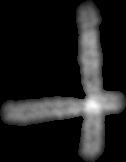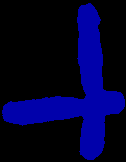## Friday, February 15, 2013

### Chromosomal shapes classification by Linear Discriminant Analysis with R

Features were tested to distinguish overlapping chromosomes from single chromosomes and from nuclei or from image segmentation artefacts:Two overlapping chromosomes
Those features are:
• The chromosomes size (normalized by the image size).
• The ratio of chromosome area by the convexhull areaconvex hull of overlapping chromosomes• The non chromosomal domains area in a convex hull. The four largest (normalized by the convex hull area) non chromosomal domains  were kept:Areas of size h1, h2, h3, h4
Chromosomes from twelve metaphases were classified with minikar. In a previous post, an isolated result indicated that these six features doesn't seem to distinguish the different particles.
Each observation falls in one of the four categories (single, cluster, nuclei, dust). 451 observations were used  to build a trained set by linear discriminant analysis (lda) according to this example.

Prior  lda, a pairs plot can be done where each color corresponds to one category (single chromosome, touching chromosomes, nuclei, dusts):Pairs plot between the six variables: var1 (area), var2 (area/convexhull), var3,4,5,6 (area of h1,h2,h3, 4)
The observations seem more or less clustered according to the pair of variables considered. The first plot (ratio ~ area) was previously done showing an overlay between the single and cluster categories. LDA was used here as a black box, with the aim to know if a good classification can be achieved and how good are the features.

Pratically the work was done with R within rstudio. Data were downloaded from github, assembled into a data frame and analysed. LDA performed here data reduction from six variables to three (LD1, LD2, LD3) and when the variable LD1 is plot against LD2, it yields:
Clearly showing separated clusters. The most populated (blue), corresponds to the single chromosomes, pink dots are the touching chromosomes, the green dots and the red dots are probably the nuclei and the dusts repectively (I have to check how to put a legend in that plot).
However, the classification is still imperfect:

28/80 touching chromosomes and 6/30 nuclei  were classified as single chromosomes. No single chromosomes were misclassified.

```# Download features and label file from github
require(RCurl)
library(RCurl)
feat_prefix <-'jp-Jpp48-'
sufix <- '-DAPI.csv'
lab_prefix <- 'shapeCateg-jp-Jpp48-'
webpath <- 'https://raw.github.com/jeanpat/pyFISH/master/GUI/karyotyper/example%20classification/source%20data/'
files <- list(1, 2, 3, 5, 6, 7, 8, 9, 10, 11, 14, 15)
data <- data.frame()
for (file in  files){
featname <- paste(webpath,'features/',sep='')
featname <- paste(featname,feat_prefix,as.character(file),sufix,sep='')
labname <- paste(webpath,'labels/',sep='')
labname <- paste(labname, lab_prefix, as.character(file),sufix,sep='')
print (featname)
print (labname)
features <- getURL(featname)
labels <- getURL(labname)
features <- read.table(text = features, sep = ';')
labels <- read.table(text = labels, sep = ';')
#print (str(features))
# add metaphase number ()
features\$metaphase <- file
#merge features and labels
features <- cbind(features,labels)
data <- rbind(data, features)
}
#print (head(data))
#remove duplicate column
data[,9] <- NULL
#rename columns
colnames(data)<-'particle'
colnames(data)<-'area'
colnames(data)<-'ratio'
colnames(data)<-'h1'
colnames(data)<-'h2'
colnames(data)<-'h3'
colnames(data)<-'h4'
colnames(data)<-'meta'
colnames(data)<-'type'
print (str(data))
pairs(cbind(data\$area,data\$ratio,data\$h1,data\$h2,data\$h3,data\$h4),col=c("red","blue","green","orange")[data\$type],upper.panel=NULL,)
#
# Linear discriminant analysis was performed as explained:
#
# http://www.youtube.com/watch?v=bOSVge_PQF4
#
library(lattice)
library(MASS)
chr.lda <- lda(type~area+ratio+h1+h2+h3+h4,data=data)
summary(chr.lda)
chr.pred <- predict(chr.lda)
ErrorTable<-table(data\$type,chr.pred\$class)
print (ErrorTable)
lda.tmp <- data.frame(chr.pred\$x,class=chr.pred\$class)
xyplot(LD1~LD2,data=lda.tmp, group=class)```
Created by Pretty R at inside-R.org